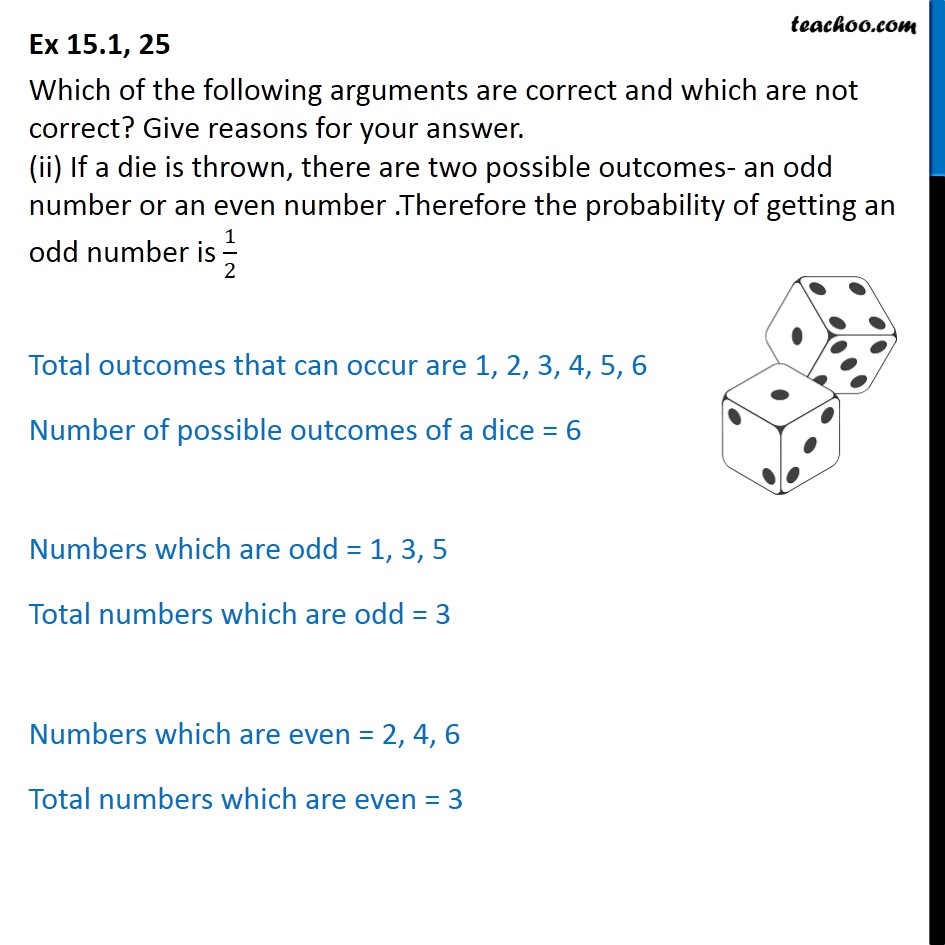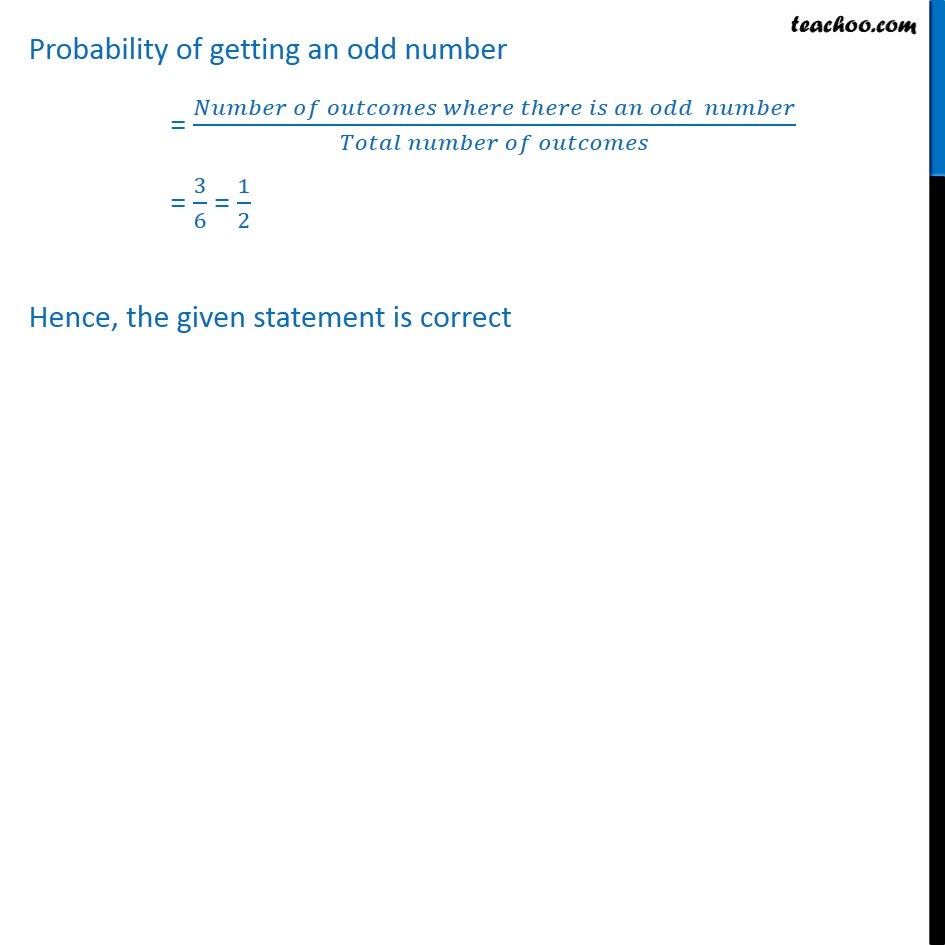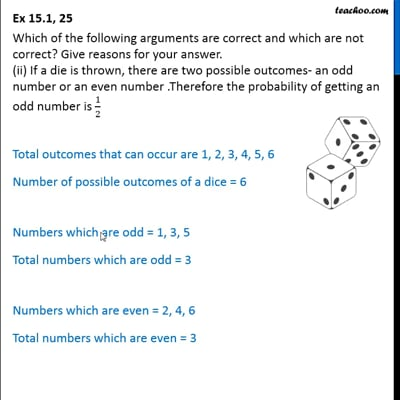Ex 15.1

Chapter 15 Class 10 Probability (Term 1)
Serial order wiseThis video is only available for Teachoo black users

### Transcript

Ex 15.1, 25 Which of the following arguments are correct and which are not correct? Give reasons for your answer. (ii) If a die is thrown, there are two possible outcomes- an odd number or an even number .Therefore the probability of getting an odd number is 1/2 Total outcomes that can occur are 1, 2, 3, 4, 5, 6 Number of possible outcomes of a dice = 6 Numbers which are odd = 1, 3, 5 Total numbers which are odd = 3 Numbers which are even = 2, 4, 6 Total numbers which are even = 3 Probability of getting an odd number = (𝑁𝑢𝑚𝑏𝑒𝑟 𝑜𝑓 𝑜𝑢𝑡𝑐𝑜𝑚𝑒𝑠 𝑤ℎ𝑒𝑟𝑒 𝑡ℎ𝑒𝑟𝑒 𝑖𝑠 𝑎𝑛 𝑜𝑑𝑑 𝑛𝑢𝑚𝑏𝑒𝑟)/(𝑇𝑜𝑡𝑎𝑙 𝑛𝑢𝑚𝑏𝑒𝑟 𝑜𝑓 𝑜𝑢𝑡𝑐𝑜𝑚𝑒𝑠) = 3/6 = 1/2 Hence, the given statement is correct